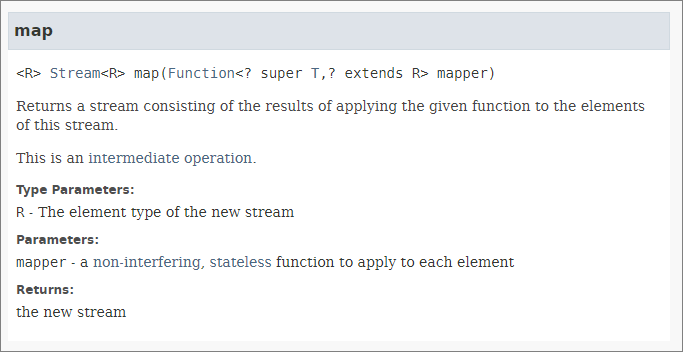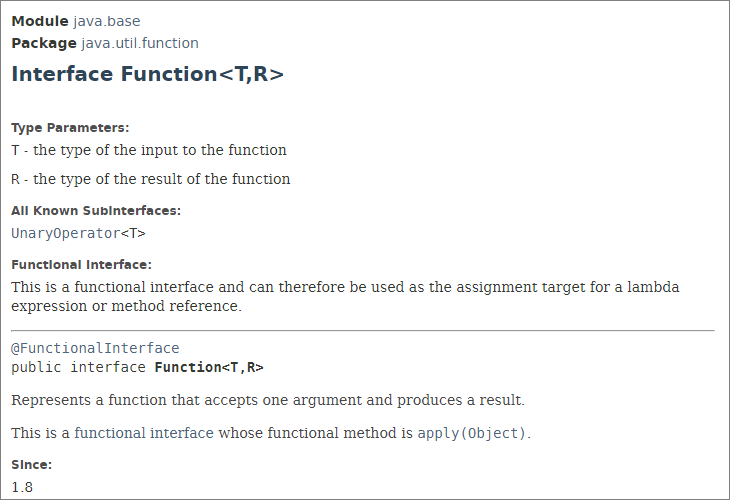Here are some notes I’ve been meaning to write up and post for a long time.The ability to perform functional-style operations on streams of elements (not to be confused with I/O streams) was introduced in Java 8, all the way back in March 2014. Combined with generic methods, (introduced in Java 5!) this provided a great deal of new flexibility - albeit with a learning curve for those of us who never spent much time studying bounded wildards.

The `Stream` interface defines the following `map()` method:That method signature has a lot going on:

`<R> Stream<R> map​(Function<? super T,​? extends R> mapper)`

A basic example implementation is this:

 `````` 1 2 3 4 5 6 7 8 9 10 11 12 13 14 `````` ``````import java.util.List; import java.util.Arrays; import java.util.stream.Collectors; public class App { public static void main(String[] args) { List letters = Arrays.asList("a", "b", "c"); List transformed = letters.stream() .map(String::toUpperCase) .collect(Collectors.toList()); } } ``````

In the above example the implementation is this:

`map(String::toUpperCase)`

How does that statement match the method signature?

Another more involved example:

 `````` 1 2 3 4 5 6 7 8 9 10 11 12 13 14 15 16 `````` ``````import java.util.List; import java.util.Arrays; import java.util.stream.Collectors; public class App { public static void main(String[] args) { List personList = ...; List students = personList.stream() .filter(p -> p.getPersonType().equals("student")) .map(p -> new Student(p.getId(), p.getName())) .collect(Collectors.toList()); } } ``````

Now, we have the following implementation of map():

`map(p -> new Student(p.getId(), p.getName()))`

Again, how does this lambda function relate to the method signature?

Looking at the overall `map()` method, we can state that it take a `Stream` of objects as its input, and generates a (presumably modified) `Stream` of (potentially different) objects as its output. The method description makes this clear - as does the `Stream<R>` return value.

Looking at the other elements of the `map()` signature, we can see take the following as a method parameter:

`Function<? super T,​? extends R> mapper`

What is this `Function` interface?This is what is responsible for transforming each input object into a related output object. For example, converting lower-case letters to upper-case letters, or creating Student objects from the relevant Person objects.

For this latter example, we might write that code as follows:

 ``````1 2 3 4 5 6 `````` ``````.map(new Function() { @Override public Student apply(Person p) { return new Student(p.getId(), p.getName()); } }) ``````

Here, we have embedded an anonymous class into the stream, in place of the lambda function we used earlier. In this case, the class is an implementation of the `Function` interface, and implements the necessary `apply()` method.

(Remember, anonymous classes are expressions, which means that you define the class inside another expression. There is no class keyword, and the class does not have a name. Anonymous classes are different from local classes, which are class declarations inside another class.)

The `Function` interface is an example of a so-called functional interface: A functional interface in Java is an interface that contains only a single abstract (unimplemented) method. (It can also contain default and static methods which do have an implementation, in addition to the single unimplemented method.)

Lambda expressions are an alternative way to implement the abstract method of a functional interface, instead of using the anonymous class shown above. When using a lambda function in this way, there is no need to include the name of the abstract method - because it is the only abstract method provided by the interface. The parameters of the lambda expression must also match the parameters of the abstract method. And the return type of the lambda expression must match as well.

(One consequence of this is that it helps to make the lambda expression as concise as possible.)

Furthermore, this explains how we can replace our anonymous class example with the lambda expression we already saw:

 ``````1 `````` ``````p -> new Student(p.getId(), p.getName()) ``````

In this lambda expression, `p` is the single input parameter - a `Person` object.

The body of the lambda expression is: `new Student(p.getId(), p.getName())`

The lambda body is only one line, and its result is therefore used as the return value - with no need for the return keyword. The return value in this case is a new `Student` object.

In our very first example, we saw this:

 ``````1 `````` ``````.map(String::toUpperCase) ``````

This is a Java method reference, which is a special type of lambda expression. It can be used here because the `toUpperCase()` method takes a single input, and produces a single output - which fits with what the `Function.apply()` abstract method expects.

That just leaves this:

`<? super T,​? extends R>`

These are bounded wildcard declarations - two of them:

`<? super T>`
`<? extends R>`

Parameterized types are invariant.  For example, `List<Type1>` is neither a subtype nor a supertype of `List<Type2>`.

`String` is a subtype of `Object`, but `List<String>` is not a subtype of `List<Object>`.

(By contrast, arrays are covariant. If `Sub` is a subtype of `Super`, then `Sub[]` is also a subtype of `Super[]`. Arrays are also reified - they know and enforce the type of the array at run-time.)

Why are parameterized types not covariant?

If you have the classes `Cat` and `Dog` which are subtypes of class `Animal`, then you can do this:

 ``````1 `````` ``````List dogs = new ArrayList<>(); // OK ``````

But you cannot then do this:

 ``````1 `````` ``````List animals = dogs; // List cannot be converted to List ``````

In the English language, a dog is an animal - and therefore a collection of dogs is also a collection of animals.

But in Java, a collection of dogs is a collection of dogs and only dogs. It is not a collection of animals.

If `List<Animal> animals = dogs;` was valid Java, then you would be able to do the following:

 ``````1 2 3 `````` ``````// NOT POSSIBLE, THANK GOODNESS: animals.add(new Cat()); // because a cat is an animal Dog dog = dogs.get(0); // How did that cat get in there? ``````

Generics only enforce their constraints at compile-time (due to type erasure).

A bounded wildcard type can provide more flexibility than invariant types.

But why - and how - is this?

Josh Bloch explains it nicely in his Effective Java book. A very high-level summary:

The expression Iterable<? extends E> means “iterable of some subtype of E” - and remembering that any type is considered a subtype of itself.

Similarly, the expression Collection<? super E> means “collection of some supertype of E” (also including E itself).

He gives the example of a generic stack, with these methods:

`void push (E e)`

and

`E pop()`

which are fine for handling individual items - but then he asks: Suppose we want to add a method that takes a sequence of elements and pushes them all onto the stack.

`void pushAll(Iterable<E>)`

The above works, as long as you are dealing with objects of type `E`. But if your objects are a subtype of `E`, then the above will fail, because parameterized types are invariant.

And also a similar `popAll(Collection<E>)` method…

The solution is our bounded wildcards. This is how Java provides a way around the “parameterized types are invariant” restriction.

Using bounded wildcards can make your methods - your APIs - more flexible.

So, we can define this:

 ``````1 2 3 4 5 `````` ``````public void pushAll(Iterable src { for (E e : src) { push(e); } } ``````

and this:

 ``````1 2 3 4 5 `````` ``````public void popAll(Colletion src { while (!isEmpty()) { dst.add(pop)); } } ``````

When you have an API which handles typed collections, then you have exactly this situation.

Of course, most of the time (maybe all of the time), as a user of such an API, you probably never think about the bounded wildcards - you just use the API and get on with the rest of your day.

Other ways I have seen this explained:

With help from this answer on Stack Overflow…

The Function’s `map()` method produces a collection of objects. Using `<? extends R>` means that the objects in the collection can be a subtype of R.

The reasoning is that a `Collection<? extends Thing>` could hold any subtype of `Thing`, and thus each element will behave as a `Thing` when you perform your operation. (You actually cannot add anything to a `Collection<? extends Thing>`, because you cannot know at runtime which specific subtype of `Thing` the collection holds.)

Conversely, when reading values from the input stream, the collection being consumed can contain supertypes of type `T`.

The reasoning here is that unlike `Collection<? extends Thing>`, `Collection<? super Thing>` can always hold a `Thing` no matter what the actual parameterized type is. Here you don’t care what is already in the list as long as it will allow a Thing to be added; this is what `? super Thing` guarantees.

Final note: The PECS mnemonic = Producer : Extends;  Consumer : Super.

“PECS” is from the point of view of the collection - the one being passed into or out of the API. If you are only pulling items from a generic collection, it is a producer and you should use `extends`; if you are only stuffing items in, it is a consumer and you should use `super`. If you do both with the same collection, you shouldn’t use either extends or super.